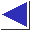# comp.lang.c FAQ list ·Question 4.9

Q: Suppose I want to write a function that takes a generic pointer as an argument and I want to simulate passing it by reference. Can I give the formal parameter type void **, and do something like this?

```	void f(void **);
double *dp;
f((void **)&dp);
```

A: Not portably. Code like this may work and is sometimes recommended, but it relies on all pointer types having the same internal representation (which is common, but not universal; see question 5.17).

There is no generic pointer-to-pointer type in C. void * acts as a generic pointer only because conversions (if necessary) are applied automatically when other pointer types are assigned to and from void *'s; these conversions cannot be performed if an attempt is made to indirect upon a void ** value which points at a pointer type other than void *. When you make use of a void ** pointer value (for instance, when you use the * operator to access the void * value to which the void ** points), the compiler has no way of knowing whether that void * value was once converted from some other pointer type. It must assume that it is nothing more than a void *; it cannot perform any implicit conversions.

In other words, any void ** value you play with must be the address of an actual void * value somewhere; casts like (void **)&dp, though they may shut the compiler up, are nonportable (and may not even do what you want; see also question 13.9). If the pointer that the void ** points to is not a void *, and if it has a different size or representation than a void *, then the compiler isn't going to be able to access it correctly.

To make the code fragment above work, you'd have to use an intermediate void * variable:

```	double *dp;
void *vp = dp;
f(&vp);
dp = vp;
```
The assignments to and from vp give the compiler the opportunity to perform any conversions, if necessary.

Again, the discussion so far assumes that different pointer types might have different sizes or representations, which is rare today, but not unheard of. To appreciate the problem with void ** more clearly, compare the situation to an analogous one involving, say, types int and double, which probably have different sizes and certainly have different representations. If we have a function

```	void incme(double *p)
{
*p += 1;
}
```
then we can do something like
```	int i = 1;
double d = i;
incme(&d);
i = d;
```
and i will be incremented by 1. (This is analogous to the correct void ** code involving the auxiliary vp.) If, on the other hand, we were to attempt something like
```	int i = 1;
incme((double *)&i);	/* WRONG */
```
(this code is analogous to the fragment in the question), it would be highly unlikely to work.